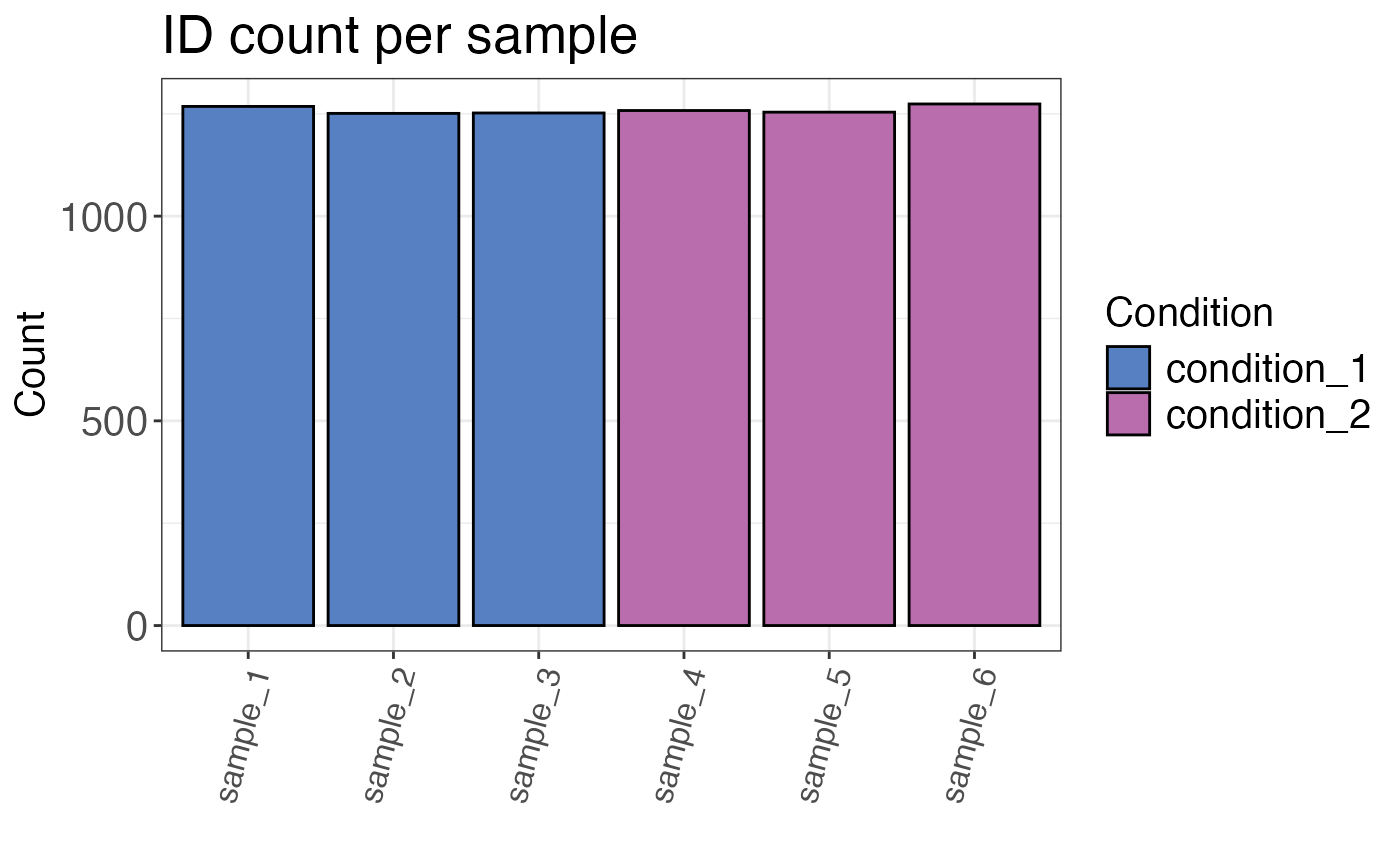Returns a plot or table of the number of IDs for each sample. The default settings remove grouping variables without quantitative information (intensity is NA). These will not be counted as IDs.

qc_ids(
data,
sample,
grouping,
intensity,
remove_na_intensities = TRUE,
condition = NULL,
title = "ID count per sample",
plot = TRUE,
interactive = FALSE
)

## Arguments

data a data frame containing at least sample names and precursor/peptide/protein IDs. a character column in the data data frame that contains the sample name. a character column in the data data frame that contains either precursor or peptide identifiers. a character column in the data data frame that contains raw or log2 transformed intensities. If remove_na_intensities = FALSE, this argument is optional. a logical value that specifies if sample/grouping combinations with intensities that are NA (not quantified IDs) should be dropped from the data frame. Default is TRUE since we are usually interested in the number of quantifiable IDs. optional, a column in the data data frame that contains condition information (e.g. "treated" and "control"). If this column is provided, the bars in the plot will be coloured according to the condition. optional, a character value that specifies the plot title (default is "ID count per sample"). a logical value that indicates whether the result should be plotted. a logical value that specifies whether the plot should be interactive (default is FALSE).

## Value

A bar plot with the height corresponding to the number of IDs, each bar represents one sample (if plot = TRUE). If plot = FALSE a table with ID counts is returned.

## Examples

set.seed(123) # Makes example reproducible

# Create example data
data <- create_synthetic_data(
n_proteins = 100,
frac_change = 0.05,
n_replicates = 3,
n_conditions = 2,
method = "effect_random"
)

# Calculate number of identifications
qc_ids(
data = data,
sample = sample,
grouping = peptide,
intensity = peptide_intensity_missing,
condition = condition,
plot = FALSE
)
#> # A tibble: 6 × 3
#>   condition   sample   count
#>   <chr>       <chr>    <int>
#> 1 condition_1 sample_3  1252
#> 2 condition_2 sample_4  1258
#> 3 condition_2 sample_5  1254
#> 4 condition_2 sample_6  1274
#> 5 condition_1 sample_2  1251
#> 6 condition_1 sample_1  1268
# Plot number of identifications
qc_ids(
data = data,
sample = sample,
grouping = peptide,
intensity = peptide_intensity_missing,
condition = condition,
plot = TRUE
)